#### Editor’s note: This month’s RF/MW boards, components and systems issue considers all off-chip (non-MMIC/RFIC) circuit design. Since this type of design involves discrete component integration and component interconnect and matching circuit design, meas...

February 5, 2011The automated load pull was introduced by Maury Microwave Corp. based on an automated slide-screw tuner in 1987. At least 20 years earlier, manual mechanical tuners of various forms were used to match transistor impedances when characterizing and designing amplifiers. Today, the importance of properly matching a transistor module when designing an amplifier is common knowledge; it is essential to use impedance matching networks on the input and output of a transistor in order to maximize power transfer, output power, gain and efficiency. The technique used to determine ideal matching network impedances is referred to as 'load pull'. This paper looks at some of the shortcomings of the older traditional methods and the improved accuracy introduced by techniques developed around the new class of nonlinear-based vector analyzers.Figure 1 Two-port scattering parameter model.

The goal of load pull is to present a set of controlled source and load impedances to the device under test (DUT) while measuring a multitude of parameters at each point. By varying the impedance, it is possible to characterize the performance of a device and design the ideal matching network for optimum realistic large-signal operating conditions.

The impedance presented to the DUT can be stated in various formats: Impedance Zload (consisting of R±jX), voltage standing wave ratio (VSWR) (as a complex number in magnitude and phase) and reflection coefficient ΓL (as a complex number in magnitude and phase). Considering the DUT as a two-port device shown in Figure 1, the magnitude of reflection presented to the device, ΓL, is nothing more than a2/b2, or the ratio between the reflected- and forward-traveling waves. The generalized formula can be written as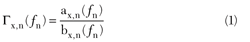From a system perspective, there are four impedances that affect our load pull measurements, as shown in Figure 2: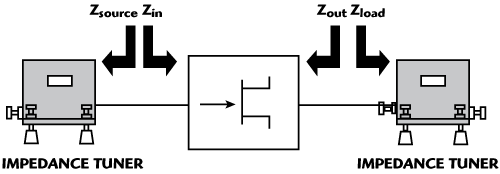Figure 2 Impedances of and presented to the DUT.

Zsource, the impedance looking from the DUT input into the source tuner and beyond

Zin, the large-signal input impedance of the DUT

Zout, the large-signal output impedance of the DUT

Zload, the impedance looking from the DUT output into the load tuner and beyond

It is the value of these impedances that determine the amount of power delivered to and reflected from the device. While Zin and Zout are characteristics of the device itself and cannot be controlled directly, the load pull system is used to vary Zsource and Zload.A traditional load pull system (see Figure 3) comprises a signal input path consisting of signal source and amplifier, source and load impedance tuners, and scalar measurement instruments such as mandatory power meters and an optional spectrum analyzer. In this type of system, the input and output powers of the DUT are determined by de-embedding the measured power from the power meters through the RF component chains and through the tuners.

Power meters are wideband in nature and measure the entire signal outputted from a transistor including fundamental and harmonic power. A highly compressed device can output significant second and third harmonic powers; however, there is no way of knowing the percentage of power allocated from each frequency. Therefore, the value read from the power meter is attributed entirely to the fundamental frequency and artificially increases the power assumed to come from the DUT. The only solution is to add a spectrum analyzer to the system which increases the cost and complexity, and it still relies on accurate de-embedding from the DUT to the instrument. In addition, these meters require considerable settling time to acquire an accurate power reading.A vector-receiver load pull system (see Figure 4) comprises a signal input path consisting of a signal source and amplifier, source and load impedance tuners, and a vector-receiver. The vector-receiver uses only the a- and b-waves calibrated at the DUT reference plane to determine measurement parameters. In this case, the a- and b-waves are analyzed on a per-frequency basis, so that each frequency component is accurately separated and used to calculate independent fundamental and harmonic powers. Additionally, a network analyzer is inherently a more accurate tool for measuring power than a power meter or spectrum analyzer.

By measuring a1, b1, a2, b2 and the instantaneous large-signal Zsource, Zin, Zout and Zload, we can more accurately calculate delivered power, gain and efficiency. The output power delivered by the device is represented asThe input power delivered to the DUT at the tuned source impedance isIn a traditional load pull system, the input impedance of the DUT is not known and the source tuner will be used to best match the input of the device in order to maximize power transfer. However, unless the source tuner is exactly matched to the complex conjugate of S11, which varies with input power, full power transfer will not occur. Therefore, the delivered power to the device will not be known and the available power will be used to determine parameters such as transducer gain and compression. The offset in the input power, gain and efficiency is caused by the mismatch between the source tuner and the device input impedance. The available input power used by the traditional system is represented as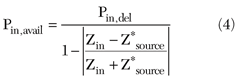It is easy to see that the available and delivered input powers converge as Zin = Z*source.

Likewise, the power gain measured in a vector-receiver load pull system is represented aswhereas in a traditional load pull system the transducer gain is represented as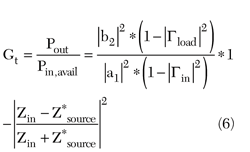whereas in a traditional load pull system the efficiency is represented asAs with input power, power and transducer gains converge and power-added and standard efficiencies converge as Zin = Z*source.

### LARGE-SIGNAL INPUT IMPEDANCE AND GAIN

A common sequence for traditional load pull is to apply a fixed signal-source power and tune the source impedance for maximum gain. The load impedance is then tuned for some maximum parameter, output power or efficiency, for example. Source and load tuning iterations continue until the optimal impedances are determined, after which, a power sweep is often performed to measure the gain compression of the device.

A DUT's large-signal input impedance varies with source power; therefore, the source impedance selected when performing source pull was optimal at only one specific power and not over the entire range of the power sweep, resulting in sub-optimal matching under the majority of conditions.Figure 5 Large-signal input impedance of DUT and three tuned source impedances.

To illustrate, Figure 5 shows the large-signal input impedance of a GaAs FET plotted as a function of power on the Smith Chart. In this case, the input impedance varies significantly with power. In the same example, three source impedances are tuned one at a time, and a power sweep is performed for each one (see Figure 6). Since the traditional load pull system is only capable of measuring available input power, the level of mismatch between the actual device input impedance and the tuned source impedance will affect the overall transducer gain of the device and differ at each power level.Figure 6 Power sweeps at each of the three tuned impedances (three separate Gt sweeps).

Because the vector-receiver load pull system measures the a- and b-waves in real time at the DUT reference plane, delivered input power is always known even if it is physically impossible to match the tuned source and DUT input impedances. The power gain Gp power sweep shows a significant improvement over transducer gain Gt power sweeps and gives a more realistic understanding of the capabilities of the DUT (see Figure 7). Transducer gain can still be used to verify the performance of the device at specific source impedances.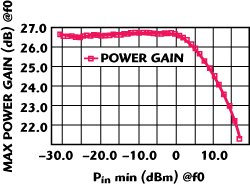Figure 7 Power sweep while matching large-signal input impedance and source impedance (one Gp sweep.

### SOURCE IMPEDANCE MATCHING

Whereas the traditional system requires actual source pull in order to visualize source contours for power and gain, vector-receiver load pull is able to mathematically compute contours. Knowing the large-signal input impedance of the device, it is possible to calculate source contours by comparing the mismatch between the proposed source impedance and the real-time large-signal input impedance, which changes as a function of power and tuned load impedance, as well as the actual input power drive (see Figure 8). Measured source-pull contours and mathematically computed source contours have been compared with excellent agreement. The ability to virtually vary the source impedance seen by the DUT and compute contours eliminates the need for multiple source pull load pull iterations thereby significantly reducing measurement time.Figure 8 Mathematically computed source contours for gain.

### TUNER CHARACTERIZATION AND DE-EMBEDDING

In a traditional load pull system, the most important factor in system accuracy is the characterization of each tuner at every frequency of interest. Characterization entails moving the tuner's internal RF probe (slug) to a multitude of horizontal and vertical positions and recording the associated S-parameters. These S-parameters are then used to calculate the loss through the tuner for power de-embedding and to calculate the impedance presented to the DUT. In order to improve the tuning accuracy, hundreds or thousands of individual tuner states are characterized. Interpolation is helpful in reducing the number of characterized states, but there are those who still insist on lengthy characterization procedures. Long-term tuner repeatability is paramount, as the S-parameters associated with each state are not re-measured.

In a vector-receiver load pull system, the impedances presented to the DUT are measured in real time. In this case, it is not important to fully pre-characterize the source and load tuners and only a small selection of points is needed, if at all. Likewise, tuner repeatability does not play a role in system accuracy as the impedances presented by the tuners to the DUT are constantly being measured. Power is determined from the a- and b-waves calibrated at the DUT reference plane, eliminating the need for tuner de-embedding.

### SYSTEM VERIFICATION

In traditional load pull systems, post-calibration system verification in the form of ΔGt or complex conjugate matched verification is critical. ΔGtcompares the theoretical gain and the measured gain at any set of source and load impedances. For this measurement, an ideal or lossy THRU with an associated S-parameter file is used as the DUT. The input and/or output impedances presented to the DUT are adjusted and the gain is both calculated from known pre-characterized S-parameters and measured with the power meter and de-embedded to the DUT reference plane. Theoretically, the calculated and measured gains should match, resulting in a ΔGt=0. However, a realistic variance up to ±0.4 dB is common across the Smith Chart.

Complex conjugate matched verification involves tuning the source and load tuners to positions that will present a conjugate match to the DUT, and measuring the gain on a THRU. The theoretical gain should be Gt=0 since the THRU is a lossless passive component, and the input and output impedances presented to the DUT are conjugate matched to eliminate mismatch losses. However, a realistic gain up to ±0.4 dB is common across the Smith Chart.

In a vector-receiver system, complex conjugate matched verification may be performed but is not essential since the impedances presented to the DUT and the powers measured from the DUT are calculated from the a- and b-waves calibrated at the DUT reference plane and not de-embedded through tuners. Verification is achieved by tuning the load tuner to some load impedance Zload and comparing it to the measured Zin on a THRU. Since the THRU line is transparent, Zin of the THRU should be equal to Zload. Verification is also achieved by measuring the actual power gain at any combination of source and load impedances on a THRU, with expectant power gain of Gp=0. Gain of up to ±0.2 dB is possible at highest tuned gammas, and can be greatly reduced by increasing the directivity of the low-loss couplers placed between the tuners and DUT.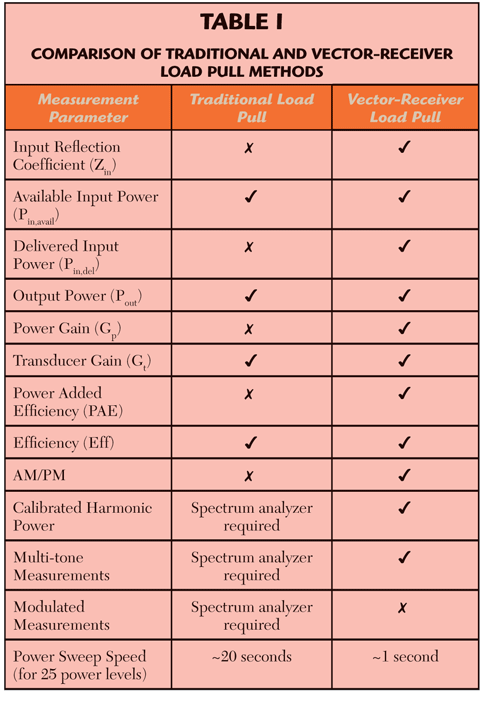### CONCLUSION

Traditional automated load pull has been used for over 20 years and is still a widely accepted method of a device characterization for amplifier design; however, it comes with the inherent weakness of not being able to accurately measure a device's large-signal input impedance. Without this device characteristic, it is impossible to accurately measure delivered input power, power gain and power-added efficiency. Because powers are measured from power meters and de-embedded through tuners, extremely accurate tuner characterization and tuner repeatability are required. Finally, multiple source-pull load pull iterations are required to converge on the optimal matching network source and load impedances. Table 1 compares the capabilities and achievable measurement parameters between traditional and vector-receiver load pull methods.

Vector-receiver load pull overcomes these weaknesses by directly measuring the a- and b-waves of a device in real-time, thereby determining the large-signal input impedance at each input power and enabling the determination of delivered input power, power gain and power-added efficiency. Since the system is calibrated at the DUT reference plane, inaccuracies arising from tuner de-embedding, and possibly lengthy tuner characterizations are eliminated. Additionally, overall measurement time is greatly reduced by the system's ability to mathematically compute source contours and eliminate the multiple source-pull load pull iterations required by traditional load pull.